Try NerdPal! Our new app on iOS and Android

# Factor the expression $x^3-3x^2-4x+12$

## Related Videos

Go!
Go!
1
2
3
4
5
6
7
8
9
0
a
b
c
d
f
g
m
n
u
v
w
x
y
z
.
(◻)
+
-
×
◻/◻
/
÷
2

e
π
ln
log
log
lim
d/dx
Dx
|◻|
θ
=
>
<
>=
<=
sin
cos
tan
cot
sec
csc

asin
acos
atan
acot
asec
acsc

sinh
cosh
tanh
coth
sech
csch

asinh
acosh
atanh
acoth
asech
acsch

### Videos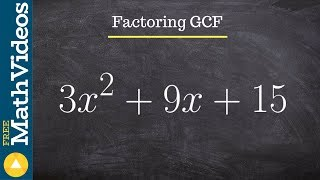### Tutorial How do you factor the GCF from an expression, 3x+6; 3x^2 +9x+15; 3x^2 y^3 -4xy^3 +5x^4 y^3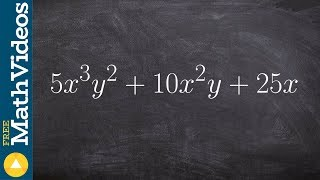### Algebra 1 - Factoring three terms with distributive property 5x^3 y^2 + 10x^2y + 25x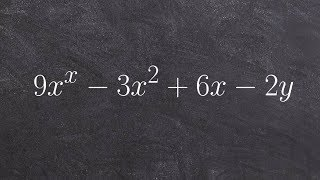### Tutorial - Factor an expression by using grouping, 9x^2 - 3xy + 6x - 2y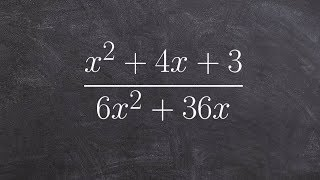### Simplify a rational expression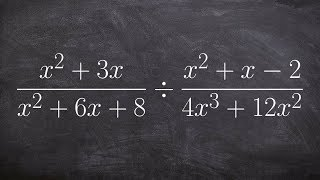### Tutorial - Simplifying by factoring to mulitply two rational expressions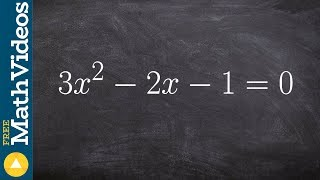SnapXam A2

### beta Got another answer? Verify it!

Go!
1
2
3
4
5
6
7
8
9
0
a
b
c
d
f
g
m
n
u
v
w
x
y
z
.
(◻)
+
-
×
◻/◻
/
÷
2

e
π
ln
log
log
lim
d/dx
Dx
|◻|
θ
=
>
<
>=
<=
sin
cos
tan
cot
sec
csc

asin
acos
atan
acot
asec
acsc

sinh
cosh
tanh
coth
sech
csch

asinh
acosh
atanh
acoth
asech
acsch

$factor\left(x^3-3x^2-4x+12\right)$